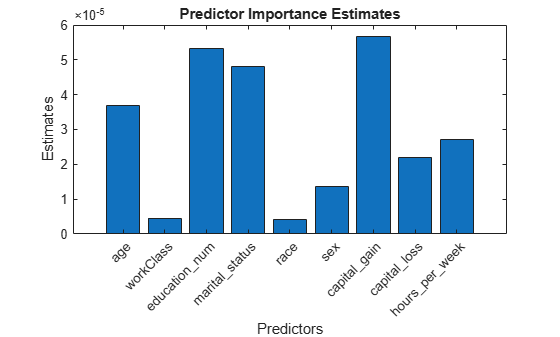Documentation

# predictorImportance

Estimates of predictor importance for classification tree

## Syntax

```imp = predictorImportance(tree) ```

## Description

`imp = predictorImportance(tree)` computes estimates of predictor importance for `tree` by summing changes in the risk due to splits on every predictor and dividing the sum by the number of branch nodes.

## Input Arguments

 `tree` A classification tree created by `fitctree`, or by the `compact` method.

## Output Arguments

 `imp` A row vector with the same number of elements as the number of predictors (columns) in `tree``.X`. The entries are the estimates of predictor importance, with `0` representing the smallest possible importance.

## Examples

expand all

`load fisheriris`

Grow a classification tree.

`Mdl = fitctree(meas,species);`

Compute predictor importance estimates for all predictor variables.

`imp = predictorImportance(Mdl)`
```imp = 1×4 0 0 0.0907 0.0682 ```

The first two elements of `imp` are zero. Therefore, the first two predictors do not enter into `Mdl` calculations for classifying irises.

Estimates of predictor importance do not depend on the order of predictors if you use surrogate splits, but do depend on the order if you do not use surrogate splits.

Permute the order of the data columns in the previous example, grow another classification tree, and then compute predictor importance estimates.

```measPerm = meas(:,[4 1 3 2]); MdlPerm = fitctree(measPerm,species); impPerm = predictorImportance(MdlPerm)```
```impPerm = 1×4 0.1515 0 0.0074 0 ```

The estimates of predictor importance are not a permutation of `imp`.

`load fisheriris`

Grow a classification tree. Specify usage of surrogate splits.

`Mdl = fitctree(meas,species,'Surrogate','on');`

Compute predictor importance estimates for all predictor variables.

`imp = predictorImportance(Mdl)`
```imp = 1×4 0.0791 0.0374 0.1530 0.1529 ```

All predictors have some importance. The first two predictors are less important than the final two.

Permute the order of the data columns in the previous example, grow another classification tree specifying usage of surrogate splits, and then compute predictor importance estimates.

```measPerm = meas(:,[4 1 3 2]); MdlPerm = fitctree(measPerm,species,'Surrogate','on'); impPerm = predictorImportance(MdlPerm)```
```impPerm = 1×4 0.1529 0.0791 0.1530 0.0374 ```

The estimates of predictor importance are a permutation of `imp`.

Load the `census1994` data set. Consider a model that predicts a person's salary category given their age, working class, education level, martial status, race, sex, capital gain and loss, and number of working hours per week.

```load census1994 X = adultdata(:,{'age','workClass','education_num','marital_status','race',... 'sex','capital_gain','capital_loss','hours_per_week','salary'});```

Display the number of categories represented in the categorical variables using `summary`.

`summary(X)`
```Variables: age: 32561x1 double Values: Min 17 Median 37 Max 90 workClass: 32561x1 categorical Values: Federal-gov 960 Local-gov 2093 Never-worked 7 Private 22696 Self-emp-inc 1116 Self-emp-not-inc 2541 State-gov 1298 Without-pay 14 NumMissing 1836 education_num: 32561x1 double Values: Min 1 Median 10 Max 16 marital_status: 32561x1 categorical Values: Divorced 4443 Married-AF-spouse 23 Married-civ-spouse 14976 Married-spouse-absent 418 Never-married 10683 Separated 1025 Widowed 993 race: 32561x1 categorical Values: Amer-Indian-Eskimo 311 Asian-Pac-Islander 1039 Black 3124 Other 271 White 27816 sex: 32561x1 categorical Values: Female 10771 Male 21790 capital_gain: 32561x1 double Values: Min 0 Median 0 Max 99999 capital_loss: 32561x1 double Values: Min 0 Median 0 Max 4356 hours_per_week: 32561x1 double Values: Min 1 Median 40 Max 99 salary: 32561x1 categorical Values: <=50K 24720 >50K 7841 ```

Because there are few categories represented in the categorical variables compared to levels in the continuous variables, the standard CART, predictor-splitting algorithm prefers splitting a continuous predictor over the categorical variables.

Train a classification tree using the entire data set. To grow unbiased trees, specify usage of the curvature test for splitting predictors. Because there are missing observations in the data, specify usage of surrogate splits.

```Mdl = fitctree(X,'salary','PredictorSelection','curvature',... 'Surrogate','on');```

Estimate predictor importance values by summing changes in the risk due to splits on every predictor and dividing the sum by the number of branch nodes. Compare the estimates using a bar graph.

```imp = predictorImportance(Mdl); figure; bar(imp); title('Predictor Importance Estimates'); ylabel('Estimates'); xlabel('Predictors'); h = gca; h.XTickLabel = Mdl.PredictorNames; h.XTickLabelRotation = 45; h.TickLabelInterpreter = 'none';```In this case, `capital_gain` is the most important predictor, followed by `education_num`.